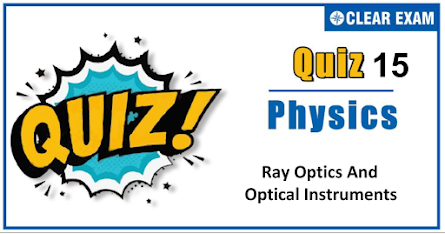## [LATEST]\$type=sticky\$show=home\$rm=0\$va=0\$count=4\$va=0

As per analysis for previous years, it has been observed that students preparing for NEET find Physics out of all the sections to be complex to handle and the majority of them are not able to comprehend the reason behind it. This problem arises especially because these aspirants appearing for the examination are more inclined to have a keen interest in Biology due to their medical background. Furthermore, sections such as Physics are dominantly based on theories, laws, numerical in comparison to a section of Biology which is more of fact-based, life sciences, and includes substantial explanations. By using the table given below, you easily and directly access to the topics and respective links of MCQs. Moreover, to make learning smooth and efficient, all the questions come with their supportive solutions to make utilization of time even more productive. Students will be covered for all their studies as the topics are available from basics to even the most advanced. .

Q1.  The spectrum of light emitted by a glowing solid is
•   Continuous spectrum
•   Line spectrum
•   Band spectrum
•   Absorption spectrum
Solution
Continuous spectrum

Q2. A lens of focal 𝑓 projects 𝑚 times magnified image of an object on a screen. The distance of the screen from the lens is
•   𝑓 /(𝑚 − 1)
•   𝑓/(𝑚 + 1)
•   𝑓(𝑚 − 1)
•   𝑓(𝑚 + 1)
Solution
Image will be real We know that 1/𝑓 = 1/𝑣 − 1/𝑢 ⇒ 𝑣/𝑓 = 1 − (𝑣/𝑢 ) (∴ 𝑢 is negative) 𝑣 = 𝑓(𝑚 + 1)

Q3.   A person uses spectacles of power + 2 D. He is suffering from
•    Myopia
•   Presbyopia
•   Astigmatism
•   Hypermetropia
Solution
Hypermetropia

Q4.  A thin plano-convex lens of focal 𝑓 is split into two halves. One of the halves is shifted along the optical axis. The separation between object and image plane is 1.8 m. The magnification of the image formed by one of the half lens is 2. Find the focal-length of the lens and separation between the two halves
•   0.1 m
•   0.4 m
•   0.9 m
•   1 m
Solution
This is a modified displacement method problem Here, 𝑎 = 1.8m and 𝑎+𝑑/𝑎−𝑑 = 2/1 Solving we get 𝑑 = 0.6 m ∴ 𝑓 = (𝑎2 − 𝑑2)/4𝑎 = 0.4m

Q5. For a concave mirror, if real image is formed the graph between 1/𝑢 and 1/𝑣 is of the form
Solution

Since 1/𝑓 = 1/𝑣 + 1/𝑢 ⇒ 1/𝑣 = − 1/𝑢 + 1/𝑓 Putting the sign convention property 1/(−𝑣) = −1/(−𝑢) + 1/(−𝑓) ⇒ 1/𝑣 = − 1/𝑢 + 1/𝑓 Comparing this equation with 𝑦 = 𝑚𝑥 + 𝑐 Slope = 𝑚 = tan𝜃 = −1 ⇒ 𝜃 = 135° or −45° and intercept 𝐶 = + 1/𝑓 .

Q6.  Rainbow is formed due to
•   Total internal reflection
•   Scattering
• Refraction
•   Dispersion and total internal reflection
Solution
The rainbow is formed due to the dispersion of white light from the sun and due to one or two total internal reflections from the water droplets behaving like prisms. The rainbow is not seen after every rain necessarily

Q7. An object of length 6 𝑐𝑚 is placed on the principal axis of a concave mirror of focal length 𝑓 at a distance of 4𝑓. The length of the image will be
•   2 𝑐𝑚
• 12 𝑐𝑚
•   4 𝑐𝑚
•   1.2 𝑐𝑚
Solution

𝐼/𝑂 = 𝑓/𝑓 − 𝑢 ⇒ 𝐼/+6 = −𝑓/−𝑓 − (−4 𝑓) ⇒ 𝐼 = −2 𝑐𝑚

Q8. A ray of light is incident on a plane mirror at an angle of 60°.The angle of deviation produced by the mirror is
•   120°
•   30°
•   60°
•   90°
Solution
Here, angle of incidence, 𝑖 = 60° ∴ Angle of deviation δ = 180° − (𝑖 + 𝑟) = 180° − 2𝑖 (As 𝑖 = 𝑟) = 180° − 2 × 60° = 60°

Q9. In a simple microscope, if the final image is located at infinity then its magnifying power is

•   25/𝑓
•   D/26
•   f/25
•   f/(D+1)
Solution
𝐷/𝐹 or 25/𝐹

Q10.  The radius of curvature of convex surface of a thin plano-convex lens is 15 𝑐𝑚 and refractive index of its material is 1.6. The power of the lens will be
•   +1 𝐷
•   −2 𝐷
•   +3 𝐷
•   +4 𝐷
Solution
𝑓 = 𝑅/(𝜇 − 1) = 15/(1.6 − 1) = 25 𝑐𝑚 ∴ 𝑃 = 100/𝑓 = 100/25 = +4𝐷## Want to know more

Please fill in the details below:

## Latest NEET Articles\$type=three\$c=3\$author=hide\$comment=hide\$rm=hide\$date=hide\$snippet=hide

Name

ltr
item
BEST NEET COACHING CENTER | BEST IIT JEE COACHING INSTITUTE | BEST NEET, IIT JEE COACHING INSTITUTE: RAY OPTICS AND OPTICAL INSTRUMENT QUIZ 15
RAY OPTICS AND OPTICAL INSTRUMENT QUIZ 15
https://1.bp.blogspot.com/-tFT7HwXN4DM/X5VN8xf13nI/AAAAAAAABi4/ElNl3StTXDMBPNPWdtsYnL7hZgSN6PdKwCLcBGAsYHQ/w445-h263/Quiz%2BImage%2B20%2B%25284%2529.png
https://1.bp.blogspot.com/-tFT7HwXN4DM/X5VN8xf13nI/AAAAAAAABi4/ElNl3StTXDMBPNPWdtsYnL7hZgSN6PdKwCLcBGAsYHQ/s72-w445-c-h263/Quiz%2BImage%2B20%2B%25284%2529.png
BEST NEET COACHING CENTER | BEST IIT JEE COACHING INSTITUTE | BEST NEET, IIT JEE COACHING INSTITUTE
https://www.cleariitmedical.com/2020/10/ray-optics-and-optical-instrument-quiz-15.html
https://www.cleariitmedical.com/
https://www.cleariitmedical.com/
https://www.cleariitmedical.com/2020/10/ray-optics-and-optical-instrument-quiz-15.html
true
7783647550433378923
UTF-8